## RD Sharma Class 8 Solutions Chapter 1 Rational Numbers Ex 1.2

These Solutions are part of RD Sharma Class 8 Solutions. Here we have given RD Sharma Class 8 Solutions Chapter 1 Rational Numbers Ex 1.2

Other Exercises

Question 1.
Verify commutativity of addition of rational numbers for each of the following pairs of rational numbers :Solution: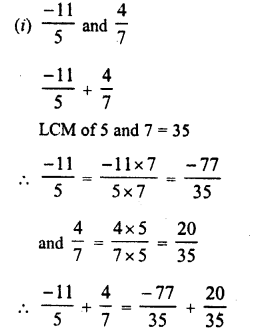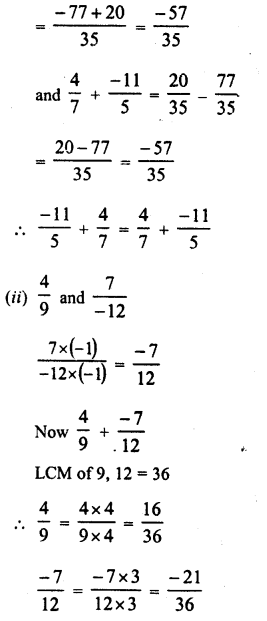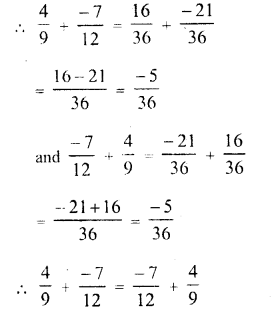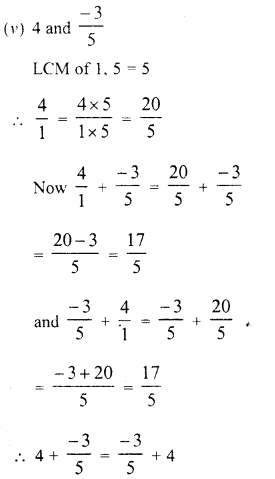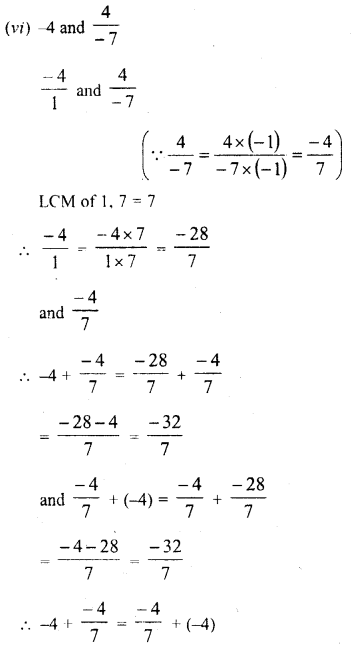Question 2.
Verify associativity of addition of rational numbers i.e., (A: + y) + z = x + (y + z), when :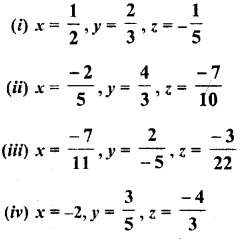Solution: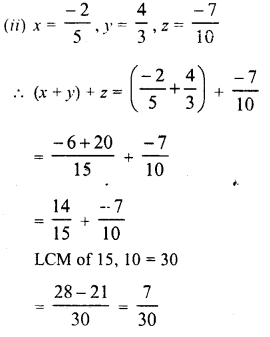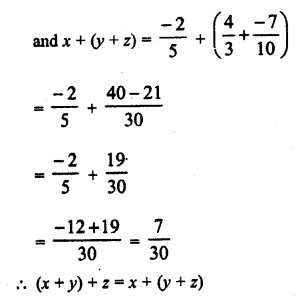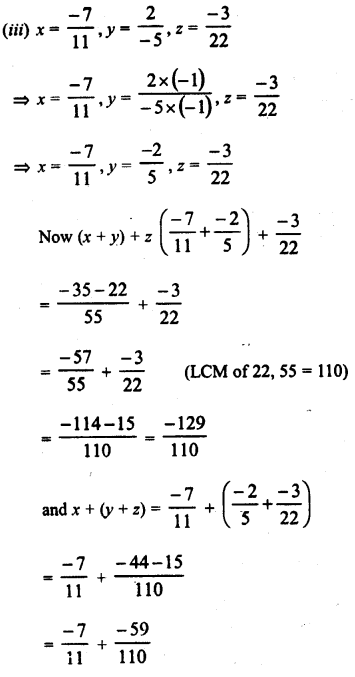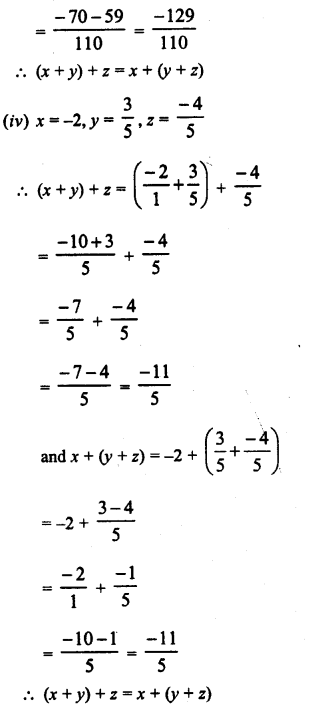Question 3.
Write the additive inverse of each of the following rational numbers :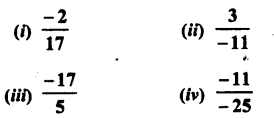Solution: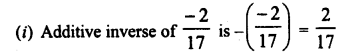Question 4.
Write the negative (additive inverse) of each of the following :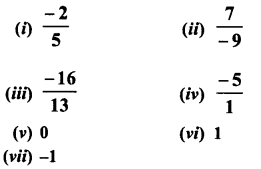Solution:Question 5.
Using commutativity and associativity of addition of rational numbers, express ‘iach of the following as a rational number :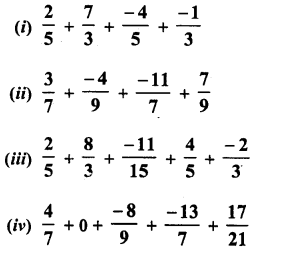Solution: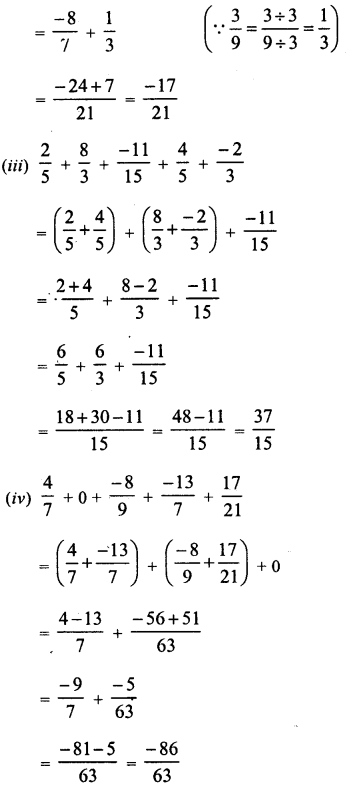Question 6.
Re-arrange suitably and find the sum in each of the following :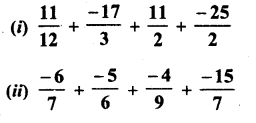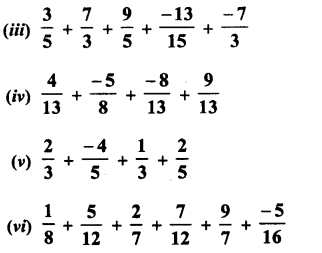Solution: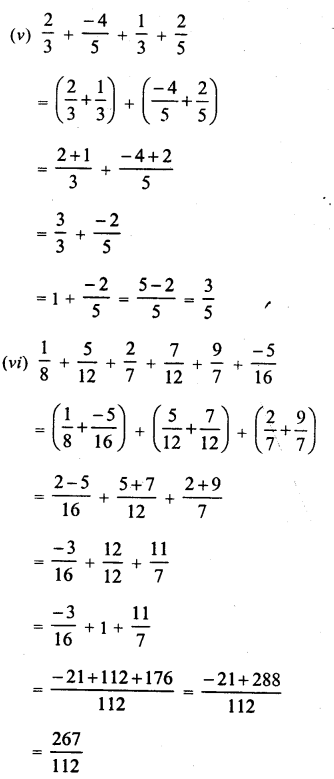Hope given RD Sharma Class 8 Solutions Chapter 1 Rational Numbers Ex 1.2 are helpful to complete your math homework.

If you have any doubts, please comment below. Learn Insta try to provide online math tutoring for you.